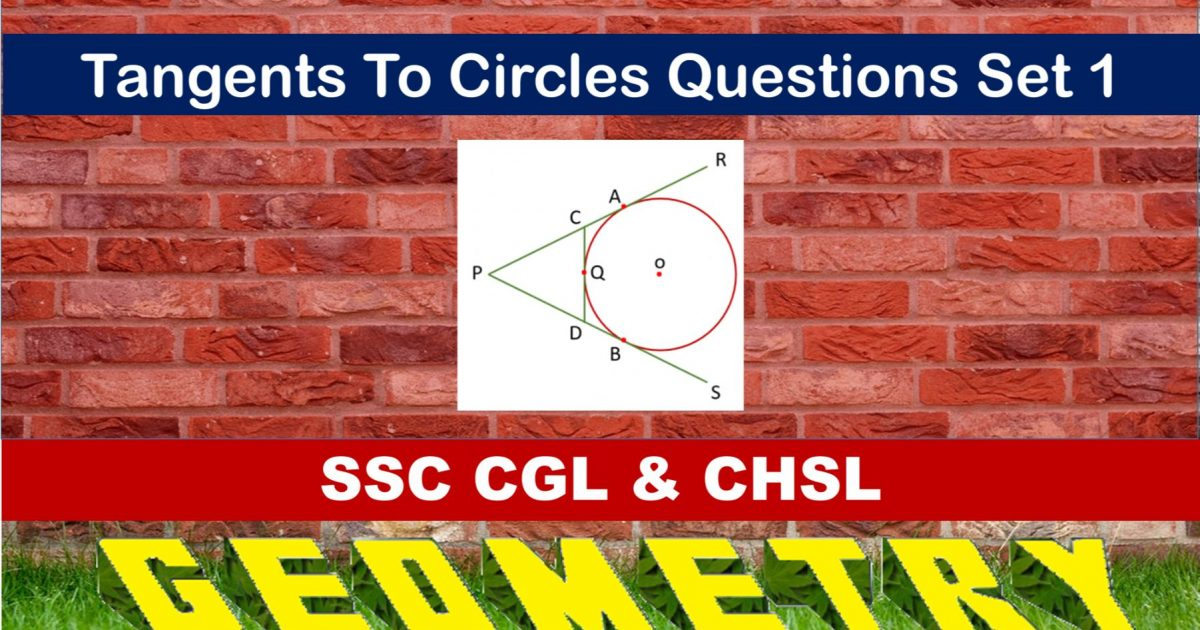Monday, September 27, 2021
Home > geometry > SSC CGL Geometry Tangent To Circle Set 1

# SSC CGL Geometry Tangent To Circle Set 1SSC CGL CHSL Geometry
Tangent to Circle Questions Sets
Set 1Set 2

SSC CGL Geometry Tangent To Circle Set 1 : Ques No 1

AB is a chord to a circle and TAP is the tangent to the circle at A. If ∠BAT = 75 degrees and ∠BAC = 45 degrees, where C is a point on the circle, then the value of ∠ABC is

Options:

A. 40 degrees

B. 60 degrees

C. 70 degrees

D. 45 degrees

Solution:

SSC CGL Geometry Tangent To Circle Set 1 : Ques No 2

If the radii of two circles be 6 cm and 3 cm and the length of the transverse common tangent be 8 cm, then the distance between the centres is

Options:

A. √140 cm

B. √145 cm

C. √135 cm

D. √150 cm

Solution:

SSC CGL Geometry Tangent To Circle Set 1 : Ques No 3

A chordAB of a circle of radius (√3 + 1) cm touches a second concentric circle of radius (√3 – 1) cm. The length of AB in cm is

Options:

A. 2∜3 cm

B. 4√3 cm

C. 8√3 cm

D. 4∜3 cm

Solution:

SSC CGL Geometry Tangent To Circle Set 1 : Ques No 4

The radii of two concentric circles are 13 cm and 8 cm. AB is a diameter of the larger circle and BD is a tangent to the smaller circle touching it at D and intersecting the larger circle at E. The length of line segment AD is

Options:

A. 17 cm

B. 18 cm

C. 19 cm

D. 20 cm

Solution:

SSC CGL Geometry Tangent To Circle Set 1 : Ques No 5

P and Q are two points on a circle with centre at O. R is the point between P and Q on the minor arc formed by P and Q. If tangents to the circle at P and Q meet at point S with ∠PSQ = 20 degrees, then ∠PQR is

Options:

A. 80 degrees

B. 100 degrees

C. 160 degrees

D. 200 degrees

Solution:

SSC CGL Geometry Tangent To Circle Set 1 : Ques No 6

A circle with centre at O touches two intersecting lines AX and BY. The two points of contact A and B subtend and angle of 65 degrees at any point C on the major arc of the circle. if P is the point of intersection of the two lines, then the measure of ∠APO is

Options:

A. 65 degrees

B. 25 degrees

C. 90 degrees

D. 40 degrees

Solution:SSC CGL Geometry Tangent To Circle Set 1 : Ques No 7

Two circles intersect at A and B. P is any point on produced BA. PT and PQ are tangents to the circle. The relation between PT and PQ is

Options:

A. PT > PQ

B. PT = PQ

C. PT < PQ

D. PT = 2PQ

Solution:

SSC CGL Geometry Tangent To Circle Set 1 : Ques No 8

AB and AC are tangents to circle. PQ another tangent of the circle at the point D on the circle, intersects AB and AC at P and Q respectively. If AB is 15 cm and QA is 9 cm, then DQ is

Options:

A. 4.5 cm

B. 3 cm

C. 7.5 cm

D. 6 cm

Solution:

SSC CGL Geometry Tangent To Circle Set 1 : Ques No 9

If two circles of radii 5 cm and 3 cm touch externally, the ratio of in which the direct common tangent to the circles divide externally the line joining the centres of the circles is

Options:

A. 2.5 : 1.5

B. 1.5 : 2.5

C. 3 : 5

D. 5 : 3

Solution:

SSC CGL Geometry Tangent To Circle Set 1 : Ques No 10

AC is the transverse common tangent to two circles with centres at P and Q and radii 6 cm and 3 cm at the points A and C respectively. If AC cuts PQ at the point B and AB is 8 cm, then the length of PQ is

Options:

A. 13 cm

B. 15 cm

C. 10 cm

D. 12 cm